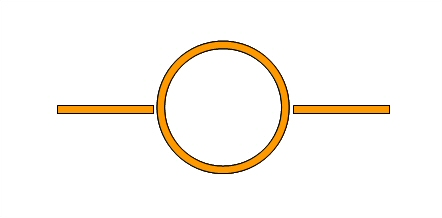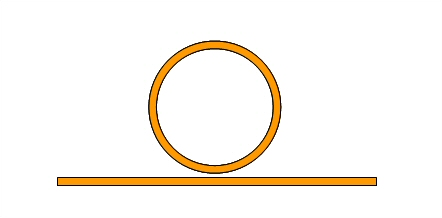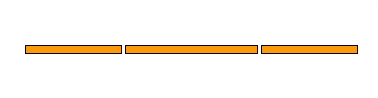# Measuring Dielectric Constant

Click here and we'll show you how to calculate dielectric constant from group delay

There are many ways to measure dielectric constant of a material or effective dielectric constant of a media. You can actually make a career out of this measurement, once you know all the nuances and practice a bit. A "complete" dielectric constant measurement include loss tangent data, which can be even harder to measure, because its effect is usually swamped by other loss mechanisms. For now let's just concentrate on obtaining accurate measurements of the real part of ER, shall we?

Before we attempt describe any methods in various amounts of detail, let's first point out that 1. dielectric constant can vary with frequency (but usually not a lot) and 2. the measurement techniques can be fraught with errors, which we'll try to explain.

Here's a true story. Someone once boasted that they had a method of measuring dielectric constant at microwave frequency that would provide three digits of resolution. We employed them to measure a single sample, and the results came back something like "6.045". The next week we asked them to perform a second sample measurement but submitted the same sample (pretty sneaky, huh?) The "new" results came back "6.267". We averaged the two data points and came up with 6.1 +/0.2! So yes indeed this measurement technique could provide three digits of accuracy, but the accuracy of the second and third digit were questionable. We told this "expert" to lose the charge number after the second sample. Moral of the story: permittivity measurements might be easy to do, but it is hard to measure permittivity with great accuracy.

The IPC (Institute for interconnecting and Packaging electronic Circuits) has documented many dielectric constant measurement technique, under IPC TM 650. Some of the techniques are at low frequencies, but some are suitable for X-band. You'll learn a lot about dielectric constant measurements by perusing IPC TM 650 section 2.5.

If you search Agilent's web site you will find app notes on this type of measurement as well.

### Dielectric constant or effective dielectric constant?

Let's mention the difference between "dielectric constant" and "effective dielectric constant". Dielectric constant is a bulk material property, effective dielectric constant is a parameter that depends on transmission line geometry. Most often the dielectric constant that engineers try to measure is the bulk measurement.

If you are considering measuring permittivity with a microstrip structure, you will be measuring the effective dielectric constant, no ifs ands or buts. If the technique involves coax, waveguide or stripline, and you are careful not to introduce appreciable air gaps or glue layers, you just might be able to directly measure the "real" dielectric constant!

### Sheet measurement technique

This is how most soft substrate suppliers measure permittivity. They merely take a very large sheet of known thickness, and measure the capacitance and back out the dielectric constant. So what's the problem? There's actually two problems. First this is (usually) low frequency (MHz, not GHz) measurement (a big sheet won't act like a lumped-element capacitor at X-band!). Again we must point out that dielectric constant may be different at microwave frequencies. The second problem is that the accuracy of the measurement is affected by the ability of the manufacturers to maintain the sample thickness; a 10% thickness tolerance equates to a potential 10% permittivity error.

### A transformer technique

If you go to our page on measuring cable lengths, there's a method that we describe as a rule of thumb for measuring cable length when you know the dielectric constant. However, you could use the same technique to measure dielectric constant of a material if you could use it to construct a long TEM transmission line, if you know the length, and if you can ensure that your measurement is done at a fifty ohm interface. We figure you could achieve 1% accuracy if you made a stripline measurement this way and included de-embedding standards.

We'll add some math and some "predicted results" to back this up one of these days. The formula is

ER=[c/(2*deltaF*L)]^2

where c is the speed of light in vacuum

deltaF is the frequency differences between two adjacent resonances

L is the known length of the transformer

(Thanks for the correction, Daniel!) Note that this doesn't give you the loss tangent, nor does it give you an ER measurement for every frequency point. It turns out with some complex math you could get all of that, plus the relative permeability. It will take us a while to develop the math into an example, if anyone wants to help us out we can supply the app note that suggests how to do this, if we can remember where we put it...

### Ring resonator techniques

There are two ways to loosely couple to a ring resonator, one is end coupling, the other is edge coupling. The end coupled structure (shown below) provides a passband whenever the ring is a multiple of wavelengths.The edge coupled technique a "suck-out" is seen in the reflection coefficient (S11) whenever the ring is an integer number of wavelengths. This is behaving like a band-reject filter, sometimes called a spur line filter (a topic we still need to cover!)

This is the preferred method if you ask us, the dips in S11 are very narrowband and therefore the resonant frequency is more accurately known.More to come!

### Coupled half-wave resonator technique

There are papers on this technique that show you how to measure both the real and imaginary parts of ER.Coming soon!

Author : Unknown Editdor